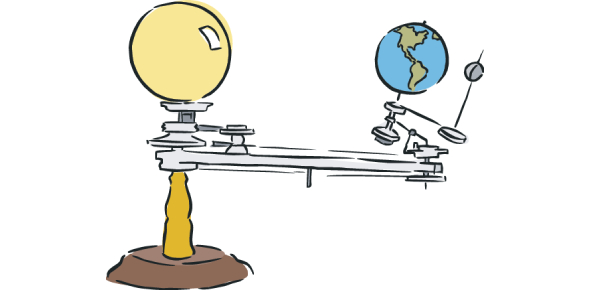# Force And Motion MCQ Exam: Quiz!

10 Questions | Total Attempts: 70Settings.

• 1.
Two sailboats are moving as shown in the graph below.
• A.

Boat A traveled faster than Boat B.

• B.

Boat B traveled faster than Boat A.

• C.

Boat A traveled for a longer time than Boat B.

• D.

Boat B traveled for a longer time than Boat A.

• 2.
A toy robot moved from a starting point and traveled in a straight line at different speeds. Then the robot turned around and traveled in a straight line at different speeds as it returned to its starting point.
• A.

The robot was moving away from the starting point.

• B.

The robot was moving toward the starting point.

• C.

The robot was stopped in one place away from the starting point.

• D.

The robot was moving back and forth around the starting point.

• 3.
Two students sit on a seesaw in a way that makes it balance and not move. When a third person pushes down on one side, that side moves down. What caused the seesaw to move?
• A.

The effect of gravity increased when one side was pushed down.

• B.

The friction between the two sides decreased because of the push.

• C.

The total force increased on the side that was pushed down.

• D.

The push on one side of the seesaw decreased the force on the other side.

• 4.
In a science lab, students measured how long it took for a block to slide down a ramp. Test Number Condition of the Ramp 1 The ramp was not covered. 2 The ramp was covered with waxed paper. 3 In the third test, the ramp was covered with sandpaper.
• A.

the amount of frictional force acting on the block

• B.

The amount of gravitational force acting on the block

• C.

the amount of frictional force acting on the ramp

• D.

The amount of gravitational force acting on the ramp

• 5.
A swimmer is moving at a speed of 2.0 meters/second. How long will it take for the swimmer to go 100 meters?
• A.

20 seconds

• B.

50 seconds

• C.

100 seconds

• D.

200 seconds

• 6.
Allen pushes a toy car across three different surfaces. He pushes the car across a wooden floor, a tile floor, and a carpeted floor. He notices it is hardest to push the toy car across the carpeted floor. Which is the MOST likely reason it is hardest to push the car across the carpet?
• A.

The car has more mass when it is on the carpeted floor.

• B.

The carpet creates more friction than the other surfaces.

• C.

The car is affected most by gravity on the carpeted floor.

• D.

The carpet pushes more against the car than the other surfaces.

• 7.
The graph shows the distance Blanca traveled for the first five minutes of her walk.
• A.

125 meters

• B.

200 meters

• C.

425 meters

• D.

500 meters

• 8.
A student is investigating the effect of force on several objects. If each ball is rolled with the same amount of force on the same surface, which ball will roll the farthest?
• A.

Golf ball

• B.

tennis ball

• C.

Soccer ball

• D.

• 9.
Ariel’s toy car uses battery power to move from one end of the track to the other. At which point on the track does gravity MOST likely increase the speed of the car?
• A.

1

• B.

2

• C.

3

• D.

4

• 10.
Which is a force that keeps the planets in orbit around the Sun?
• A.

inertia

• B.

gravity

• C.

Magnetism

• D.

Solar energy

Related TopicsBack to top